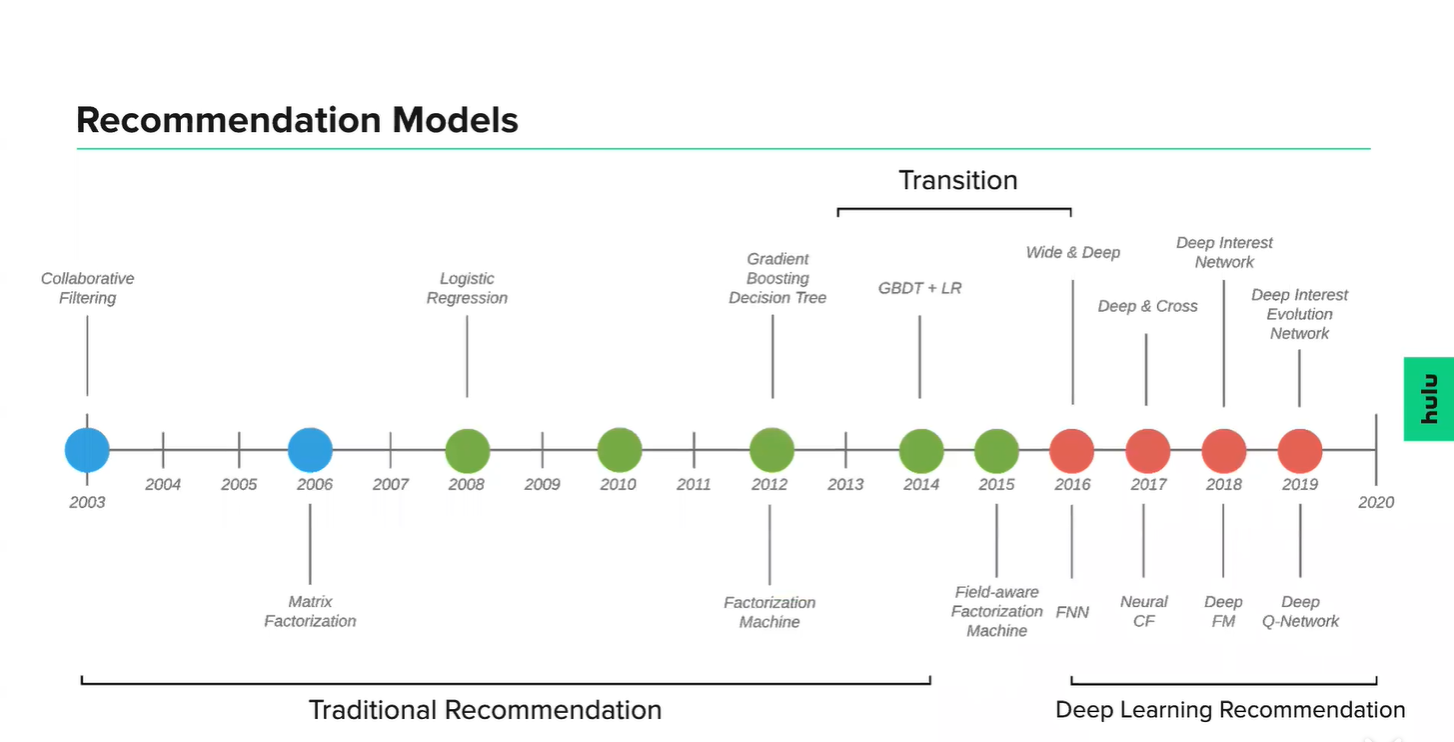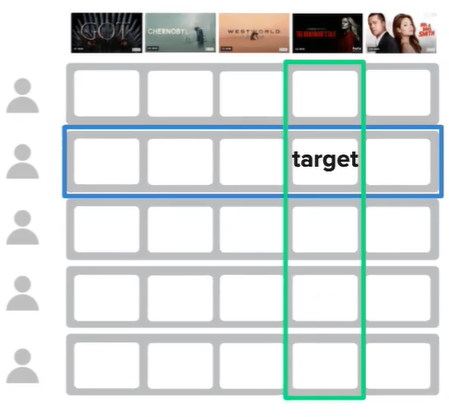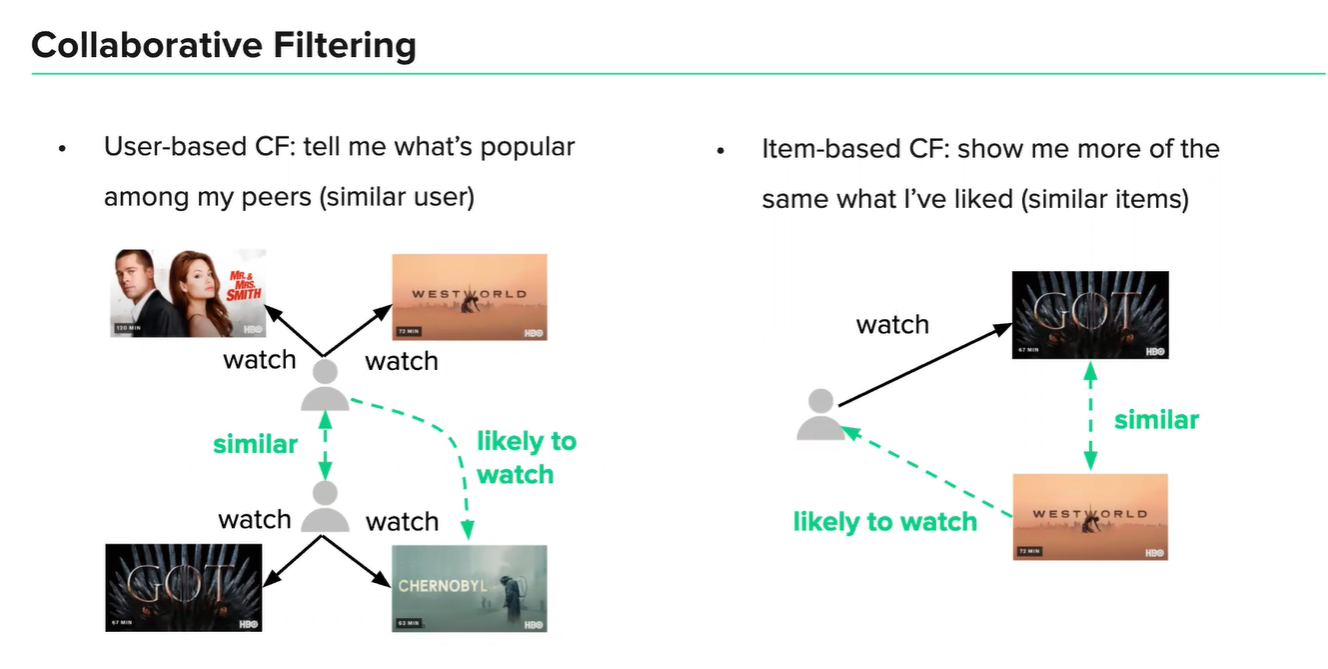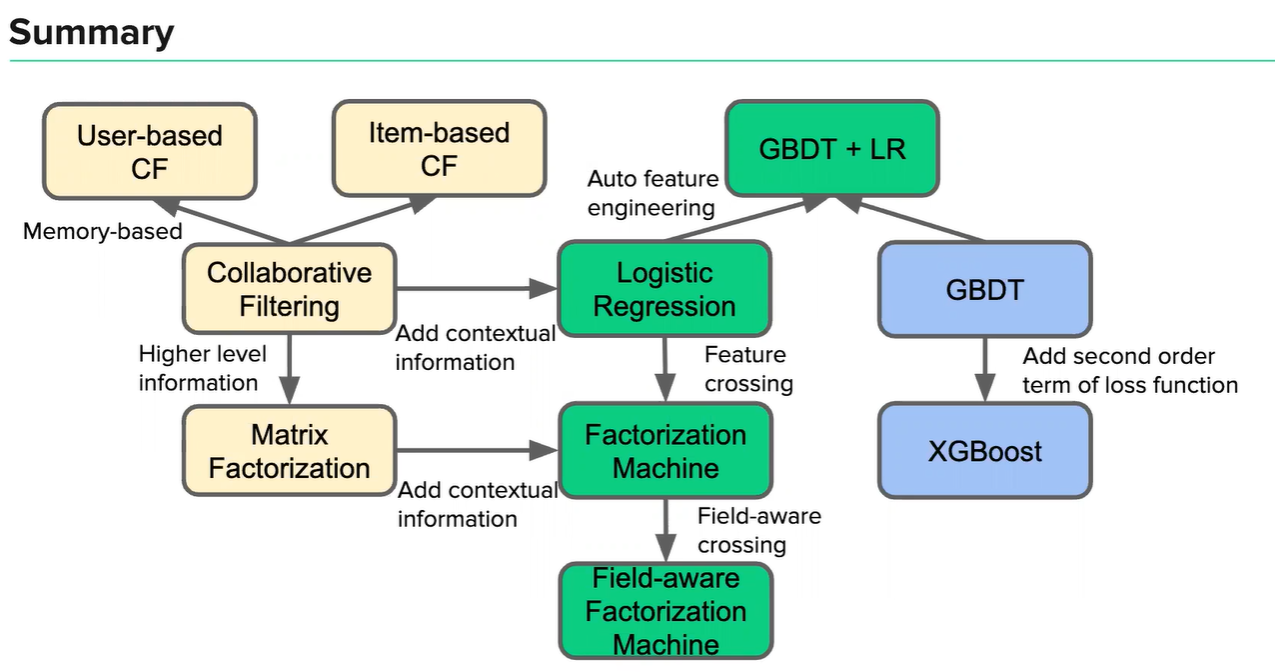# The aim of this class

• Basic concept of recommender systems
• Simple formula and theory
• Underlying intuition of each recommendation model
• Pros and cons

# What is recommender system?

Basic assumption of recommender systems

• Users are unsure about what they are looking for(different from information retrieval)

• Develop a mathematical model of an objective function($\mathcal{F}$) to predict how much a user will like an item in a given context.# Basic Concept

• Ratings

• Explicit ratings: 5 star, like/dislike(additional effort from users)
• Implicit ratings: page views, click, effective playback, purchase records, whether or not listen to a music track(easier to collect, less precise)
• Interaction matrix

• Matrix modelling user’s rating on item
• User $u$’s rating towards item $i$ as $r_{u,i}$# Collaborative Filtering “物以类聚，人以群分”

• Context-free recommender system

• Intuition: the users who have agreed in the past tend to also agree in the future

• F: aggregate from nearest neighbor

• User-based CF: user neighbors (green block)

• Item-based CF: item neighbors (blue block)

• Hybrid: use both## Similarity Calculation

• Cosine similarity
• Pearson correlation
• SimRank

## Improvement of Similarity Calculation

• Not all neighbors are equally “valuable” i.e. agreement on commonly liked items not so informative as agreement on controversial items
• Give more weight to items that have a higher variance
• Low number of co-rated items may introduce bias
• Reduce “confidence” when the number of co-rated items is low
• All neighbors are not very “similar”
• Set similarity threshold
• Set maximum number of neighbors

## Pros and Cons

• Pros

• Easy to implement
• Good explanation
• Cons

• Large Memory needed
• Sparsity issue

# Matrix Factorization

• Context-free recommender system
• Intuition: express higher-level attributes
• Generate user and item embeddings

• Latent vectors
• (A group of) similar users/items have similar embeddings

$\mathcal{F}$ : dot product of embeddings $r_{u,i}=p_u^T q_i$

## Improvement of Matrix Factorization

• $\mathcal{F}$ could be more complicated

• Global bias

• Average ratings in the whole catalog
• Item bias

• Users rate diﬀerent categories in diﬀerent ways
• User bias

• Some users tend to give high ratings while others are not

## Pros and Cons

• Pros:
• Better generalization capability: even though two users haven’t rated any same movies, it’s still possible to ﬁnd the similarity between them through embeddings
• Low memory: only need to store low-dimensional latent vectors, no need to store large user behaviors
• Cons
• Hard to explain
• Sparsity

# Logistic Regression

• Intuition: add context information to our model

• Recommendation as classiﬁcation:

• $\mathcal{F}$

• User, item, context => categorical feature vector

• Example: gender, time diﬀerence within the day, show genre as feature
• $[0, 1, 0, 0, 0, 1, 0]$ => female, view on morning, target show is Horror Movie
• Diﬀerent weight of features, learned by gradient descent

• Sigmoid projection

## Logistic Regression —— why?

• Why categorical feature vector?

• Some non-numerical features, e.g. device: “roku”, “web”, “android”…
• Absolute value of feature does not make sense
• Feature crossing
• Fast computation of sparse vector
• Why sigmoid projection?

• Output between 0 and 1
• Good mathematical form
• Maximum entropy model

## Pros and Cons

• Pros
• Good explanation (feature importance)
• Good parallelism, fast model training
• Low training cost
• Online training
• Cons
• Manually craft features
• Limited model expressiveness (linear model)

# Factorization Machine (FM)

• Intuition: feature crossing

• $\mathcal{F}$

• Let $w_{ij}=$

• Deal with parameter explosion ($n^2 \geq nk$)

• Equivalent to low rank parameter matrix factorization $W=VV^T$

## Pros and Cons

• Pros
• Good expressiveness
• Good generalization
• Relatively low training cost
• Cons
• Hard to make higher level feature crossing

# Gradient Boosting Decision Tree (GBDT)

• $\mathcal{F}$

• Training samples $(x_1, y_1), (x_2, y_2), … , (x_n,y_n)$

• Boosting:

• $f$ : Regression tre -> Boosting Regression(Decision) Tree

• Loss function -> Gradient Boosting Decision Tree, GBDT

## XGBoost & LightGBM

• $\mathcal{F}$

• Change optimization goal

• Loss function: Taylor expansion, keep second order terms

• Regularization
• Prevent too complicated trees
• Prevent extreme parameters
• LightGBM (engineering optimization)

## Pros and Cons

• Pros
• Good expressiveness
• Low eﬀort of feature engineering
• Cons
• Hard parallelism
• Unable to do incremental training

# GBDT + LR

• Intuition: model-based feature engineering